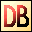DelphiBasicsShlKeyword Shift an integer value left by a number of bits
 Number Shl bits
Description
The Shl keyword performs a bitwise shift left of an Integer. The number is shifted Bits to the left.

If Bits is greater than the size of the number type, the Bits value is Mod'ed by the number type size before the shift.

For example;

var
myByte : Byte;
begin
myByte := \$2F;   // \$2F = 47 decimal
myByte := myByte Shl \$24;
end;

Gives the same result as Shl 4 = \$F0.
Notes
Warning Only use Shl when a bit operation is required - do not use instead of a multiplication or division. First because it is unclear as to what is happening. Secondly, bits may be lost in the operation.
Related commands
 Hi Returns the hi-order byte of a (2 byte) Integer Lo Returns the low-order byte of a (2 byte) Integer Shr Shift an integer value right by a number of bitsDownload this web site as a Windows program.